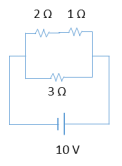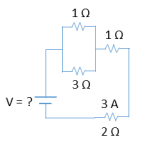Ch 25: Resistors & DC CircuitsWorksheetSee all chapters
 Ch 01: Units & Vectors 2hrs & 22mins 0% complete Worksheet Ch 02: 1D Motion (Kinematics) 3hrs & 11mins 0% complete Worksheet Ch 03: 2D Motion (Projectile Motion) 3hrs & 8mins 0% complete Worksheet Ch 04: Intro to Forces (Dynamics) 3hrs & 42mins 0% complete Worksheet Ch 05: Friction, Inclines, Systems 4hrs & 32mins 0% complete Worksheet Ch 06: Centripetal Forces & Gravitation 3hrs & 51mins 0% complete Worksheet Ch 07: Work & Energy 3hrs & 55mins 0% complete Worksheet Ch 08: Conservation of Energy 6hrs & 54mins 0% complete Worksheet Ch 09: Momentum & Impulse 5hrs & 35mins 0% complete Worksheet Ch 10: Rotational Kinematics 3hrs & 4mins 0% complete Worksheet Ch 11: Rotational Inertia & Energy 7hrs & 7mins 0% complete Worksheet Ch 12: Torque & Rotational Dynamics 2hrs & 9mins 0% complete Worksheet Ch 13: Rotational Equilibrium 4hrs & 10mins 0% complete Worksheet Ch 14: Angular Momentum 3hrs & 6mins 0% complete Worksheet Ch 15: Periodic Motion (NEW) 2hrs & 17mins 0% complete Worksheet Ch 15: Periodic Motion (Oscillations) 3hrs & 16mins 0% complete Worksheet Ch 16: Waves & Sound 3hrs & 25mins 0% complete Worksheet Ch 17: Fluid Mechanics 4hrs & 39mins 0% complete Worksheet Ch 18: Heat and Temperature 4hrs & 9mins 0% complete Worksheet Ch 19: Kinetic Theory of Ideal Gasses 1hr & 40mins 0% complete Worksheet Ch 20: The First Law of Thermodynamics 1hr & 49mins 0% complete Worksheet Ch 21: The Second Law of Thermodynamics 4hrs & 56mins 0% complete Worksheet Ch 22: Electric Force & Field; Gauss' Law 3hrs & 32mins 0% complete Worksheet Ch 23: Electric Potential 1hr & 55mins 0% complete Worksheet Ch 24: Capacitors & Dielectrics 2hrs & 2mins 0% complete Worksheet Ch 25: Resistors & DC Circuits 3hrs & 20mins 0% complete Worksheet Ch 26: Magnetic Fields and Forces 2hrs & 25mins 0% complete Worksheet Ch 27: Sources of Magnetic Field 2hrs & 30mins 0% complete Worksheet Ch 28: Induction and Inductance 3hrs & 38mins 0% complete Worksheet Ch 29: Alternating Current 2hrs & 37mins 0% complete Worksheet Ch 30: Electromagnetic Waves 1hr & 12mins 0% complete Worksheet Ch 31: Geometric Optics 3hrs 0% complete Worksheet Ch 32: Wave Optics 1hr & 15mins 0% complete Worksheet Ch 34: Special Relativity 2hrs & 10mins 0% complete Worksheet Ch 35: Particle-Wave Duality Not available yet Ch 36: Atomic Structure Not available yet Ch 37: Nuclear Physics Not available yet Ch 38: Quantum Mechanics Not available yet

# Solving Resistor Circuits

See all sections

Concept #1: Solving Resistor Circuits

Transcript

Hey guys. So, in this video we're going to talk about how to solve more complicated circuit problems, let's check it out. Alright, so in some circuit problems you'll be asked to find not just the equivalent resistance but also the current i and the voltage V of different resistors. Alright, so let's see how we do that, but first, let me remind you that resistors can be connected in series or in parallel and if they're in series, this is the equation used to find the equivalent resistance, this is the equation for equivalent resistance in parallel. Remember, that in parallel there are also two shortcut equations you can use, two shortcuts, so this is old news, what's new here is this stuff here, right? And, when you have resistors in series they will share current with each other, they will share current with each other. So, for example, if I have a 1 ohm resistor 2 and 3, 2 ohms, 3 ohms and if I know that this current here is 2 amps then I know, if I'm given the current 2 amps then I know that this here has to be 2 amps and then I know that this here also has to be 2 amps, because they're in series, the current is flowing this way, it has nowhere to go so it has to just flow with a constant 2 amps. So, they share current with each other, cool? Now, you need to learn that but you also have to remember that they share current with the equivalent resistor as well So, they share current with the equivalent resistor, what does that mean? Well, if I combine these I just add them. So, I have one, two and three, this is 6 ohm resistor, but this 2 here is not only the same for these guys but it's also the same here. So, I'm going to know that this has to be a 2 amp current going through this resistor. So, not only is the current the same with each other when you're combining in series but also with the combined merged resistor, okay? Very important.

Practice: What is current and voltage across each resistor below?Example #1: Find Current of One Resistor

Transcript

Hey guys. So, this example I want to show you how some of these problems are actually deceivingly simple, they look complicated but they're very straightforward, this is an example of them. So, in this question instead of asking for everything, we're being asked for just one thing, which is what is the current through the swinging ohm resistor which is this guy here. So, that's all we need to know, let's call this the current through the 4 ohm, what is it okay? Well, remember, the equation that connects all these variables is Ohm's law V equals i, R. So, you can find i as long as you have V and R if you solve for i, u get V over R, I know the resistance is 3 ohms. So, really all I need is the voltage. Now, you may also remember that the voltage is the same in parallel and it's not just the same between resistors it's the same for any circuit, any circuit element, so the voltage across these two points is the same through that branch is the same as the voltage across these two points and it's the same for this voltage and it's the same for the voltage between these two resistors. So, because the voltage through this battery is 5, I can say that the voltage on the 3 ohms has to be 5 as well because they're parallel. So, that's it, this voltage is 5 volts, I can divide the two and the answer is going to be 1.67, 1.67 amps and we're done, super straightforward, but you have to be able to recognize certain properties, okay? So, some of these questions are going to be very long and some of them are going to be tricky and simple. So, you gotta be ready for both, let's keep going.

Practice: What is the voltage of the battery below?